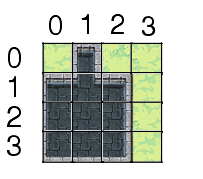# The lexicographical (or row-major) order of a map

By on

Storing a tile-based map in a JavaScript array efficiently

Take this 2-dimensional tile-based map:

[object Object]

As you can see according to these (x,y) coördinates

• (0,0) = grass
• (0,1) = water
• (0,2) = grass
• ...

Storing this map in memory can happen in two ways, for example you can store every Y-position in an X-row, like this:

``````map[X][Y] = tile;
``````

So:

• map = grass
• map = water
• map = grass
• ...

But there's a little problem in Javascript to look this up. For example, if you want to look up a certain value you would do something like this:

``````if (map !== undefined){
tile = map;
}
``````

But what if the X isn't defined, and you're directly searching for a Y value in it? It would throw an error in this case. So you actually have to do:

``````if (map !== undefined){
if (map !== undefined){
tile = map;
}
}
``````

So 2 lookups for 1 coördinate.

A better way to do it would be to store it lexicographically.

Basically, you calculate the ID of the tile, what total number it is on the map, following this formula:

### lexicograph = y * width + x

And that gives you this order:

[object Object]